Courses

# Test: Thin Cylinder Level - 1

## 10 Questions MCQ Test Solid Mechanics | Test: Thin Cylinder Level - 1

Description
This mock test of Test: Thin Cylinder Level - 1 for Mechanical Engineering helps you for every Mechanical Engineering entrance exam. This contains 10 Multiple Choice Questions for Mechanical Engineering Test: Thin Cylinder Level - 1 (mcq) to study with solutions a complete question bank. The solved questions answers in this Test: Thin Cylinder Level - 1 quiz give you a good mix of easy questions and tough questions. Mechanical Engineering students definitely take this Test: Thin Cylinder Level - 1 exercise for a better result in the exam. You can find other Test: Thin Cylinder Level - 1 extra questions, long questions & short questions for Mechanical Engineering on EduRev as well by searching above.
QUESTION: 1

### In thin cylinder, circumferential stress is x times longitudinal stress, where x is

Solution: In a thin cylinder,

Hoop stress, σh =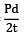longitudinal stress σl =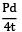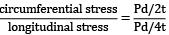= 2

QUESTION: 2

### If the cylindrical pipe is subjected to internal fluid pressure, the nature of hoop stress is

Solution: Hoop stress acts along the circumference this stress is tensile in nature.

QUESTION: 3

### A thin cylinder with closed ends is subjected to internal pressure and supported at ends as shown in figure. What is the state of stress at point x?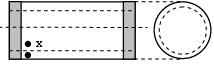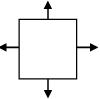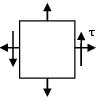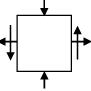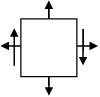Solution: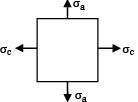No. shear stress, τ Hoop and axial stresses act, both are tensile.

QUESTION: 4

Maximum value of shear stress in thin cylinders is equal to

Solution: Max. Shear Stress

Max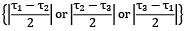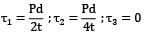τmax =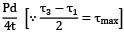=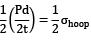QUESTION: 5

A thin cylinder with both ends closed is subjected to internal pressure p. The longitudinal stress at the surface has been calculated as σ0 . Maximum shear stress at the surface will be

Solution: At the surface σa = σ0 σc = 2σ0 P = 0 (radial stress)

τmax =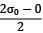= σ0

QUESTION: 6

If the hoop strain and longitudinal strain in case of a thin cylindrical shell are eh and et then volumetric strain is

Solution:
QUESTION: 7

A pipe having internal diameter d, wall thickness t and internal pressure p, the bursting pressure is

Solution:
QUESTION: 8

A thin cylinder contains fluid at a pressure of 500 MN/m2, internal diameter of the shell is 0.6 m and tensile stress in the materials is to be limited to 9000 N/m2. The shell must have a minimum thickness of nearly

Solution: σc = 9000

=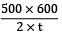t = 16.66 ≃ 17 mm

QUESTION: 9

When is the safe working pressure for a spherical pressure vessel 1.5 m internal diameter and 1.5 cm wall thickness if the maximum allowable tensile stress in vessel is 45 MPa?

Solution: σall =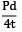P =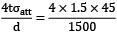= 1.8 MPa

QUESTION: 10

What is the safe working pressure for a spherical pressure vessel 1.5m internal diameter and 1.5cm wall thickness, if the maximum allowable tensile stress is 45 MPa?

Solution: Hoop stress ≤ Maximum allowable tensile stress (for spherical vessel)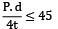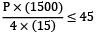P ≤ 1.8 MPa For safe working, pressure should be less than 1.8 MPa